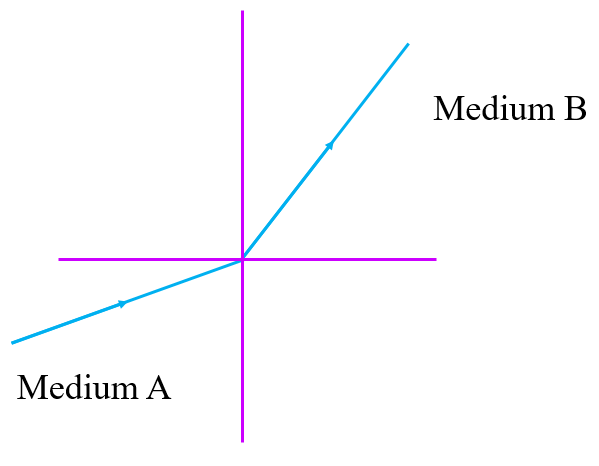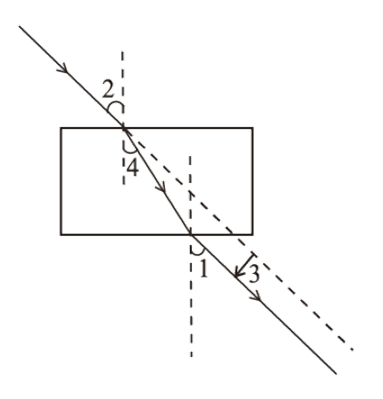# vs.eyeandcontacts.com

## Chapter 10 - Light - Reflection and Refraction Extra Questions

1. A student very cautiously traces the path of a ray through a glass slab for different values of the angle of incidence (Li). He then measures the corresponding values of the angle of the angle of refraction (Lr) and the angle of emergence (Le) for every value of the angle of angle of incidence. On analyzing these measurements of angles, his conclusion would be
a) Li > Lr > Le
b) Li = Le > Lr
c) Li < Lr < Le
d) Li = Le < Lr
b) Li = Le > Lr

2. A light ray enters from medium A to medium B as shown is the figure. The refractive index of medium B relative to A will bea) Greater than unity
b) Less than unity
c) Equal to unity
d) Zero
a) Greater than unity

3. The light changes its path as its medium changes. Which of the following is an incorrect statement?
a) Speed of light is different in different media.
b) Light changes its path because light only travels in a straight line.
c) Speed of light is dependent on medium through which it is passing.
d) The light chooses the path with minimum time, as it changes its medium.
b) Light changes its path because light only travels in a straight line.

4. What is the unit of refractive index?
a) Pascal
b) Joule
c) No unit
d) m/s
c) No unit

5. Light travels fastest in:
a) Vacuum
b) diamond
c) glass
d) turpentine
a) Vacuum

6. If the speed of light in vacuum is 3 x 10^8 m/s, find speed of light in a medium of absolute refractive index 1.5.
a) 4.5 x 10^8 m/s
b) 1.5 x 10^8 m/s
c) 0.5 x 10^8 m/s
d) 2 x 10^8 m/s
d) 2 x 10^8 m/s

7. The correct sequence of angle of incidence, angle of emergence, angle of refraction and lateral displacement shown in the following diagram by digits 1, 2, 3 and 4 is:a) 2, 4, 1, 3
b) 2, 1, 4, 3
c) 1, 2, 4, 3
d) 2, 1, 3, 4
b) 2, 1, 4, 3

8. The angle of incidence i and angle of refraction r are equal in a transparent slab when the value of angle of incidence is:
a) Zero degrees
b) 45 degrees
c) 90 degrees
d) depends on the material medium
a) Zero degrees

9. Light from the sun falling on a convex lens will converge at a point called
a) centre of curvature
c) focal length
d) Principal focus
d) Principal focus

10. Find the absolute refractive index of a medium in which light travels with a speed of 1.4 x 10^8 m/s.
a) 1.4
b) 2.14
c) 3.1
d) 4.0